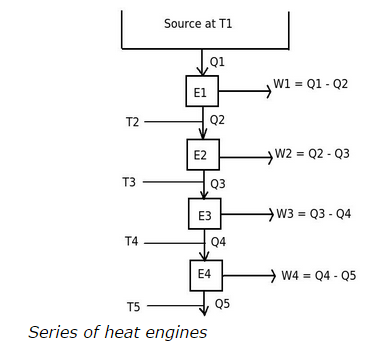# To achieve a definite zero point on the Kelvin scale, we have to violate

## To achieve a definite zero point on the Kelvin scale, we have to violate

a. first law of thermodynamics
b. second law of thermodynamics
c. both first law and second law of thermodynamics
d. no law has to be violated

Correct Answer: b. second law of thermodynamics

Explanation:

To achieve a definite zero point on the Kelvin scale or absolute zero temperature scale, we have to consider a series of reversible engines, passing from a source at T1 to lower temperatures. Consider the following diagram,The heat engine E1 is operating between temperatures T1 and T2.
Therefore,

( T1 / T2 ) = ( Q1 /Q2 )

( T1 – T2 ) / T2 = ( Q1 – Q2 ) / Q2

( T1 – T2 ) = ( Q1 – Q2 ) ( T2 / Q2 )

Similarly,

( T2 – T3 ) = ( Q2 – Q3 ) ( T3 / Q3 )
and so on

If we make this series of heat engines continue so that the total work output equals to heat supplied Q1. This means there will not be any heat rejection and definite zero point on the Kelvin scale is achieved. But as there is no any heat rejection means total heat supplied is completely converted into work output. This condition violates the second law of thermodynamics.